# Of gravitational waves and spherical chickens

### Information about a class of simple model universes, each an expanding cosmos filled with gravitational waves

#### An article by Robert H. Gowdy

An endless series of physics jokes have a punch line that begins “Assume a spherical chicken….” These jokes poke fun at the way that physicists think, but make a serious point. Often an extreme simplification leads to a situation that can be understood completely with just pencil and paper and reveals trends that might have been missed among the details of a fully realistic calculation. One real situation that we are interested in understanding is the early universe, and one simplification to help our understanding of certain aspects thereof are the so-called Gowdy spacetimes.

## Gravitational waves in the early universe

The time is just after the initial big bang singularity. Gravitational waves are everywhere, with all sorts of wavelengths, headed in all directions. The extreme simplification of this situation is to assume the following:

1. No matter is present.
2. All of the gravitational wave fronts are parallel to each other and do not twist.
3. The same wave pattern repeats over and over again in space.

The first assumption is easy to understand. The second one can be understood as follows: As gravitational waves move through space, at any given moment some regions of space will experience maximum distortion (see the spotlight text The wave nature of gravitational waves). For the simple waves we are looking at here, these regions of maximal contortion are planes. As the wave pattern repeats, these regions are stacked one above the other somewhat like this: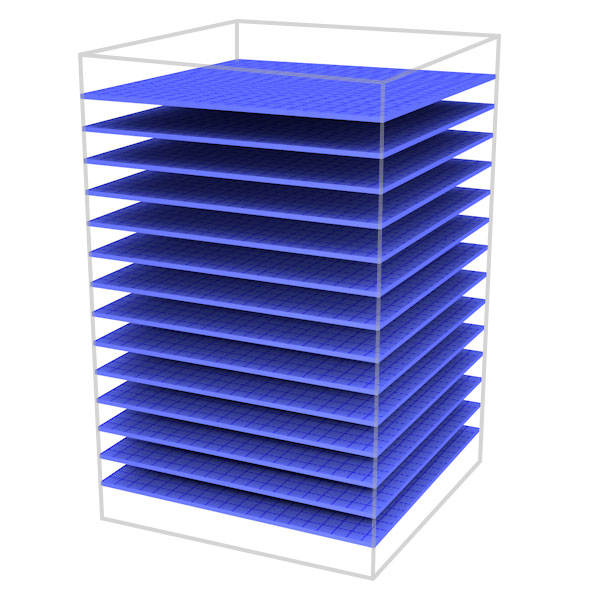The extra assumption that these planes do not twist means that they are not twisted like a stack of cards with each card rotated at an angle to the one below it: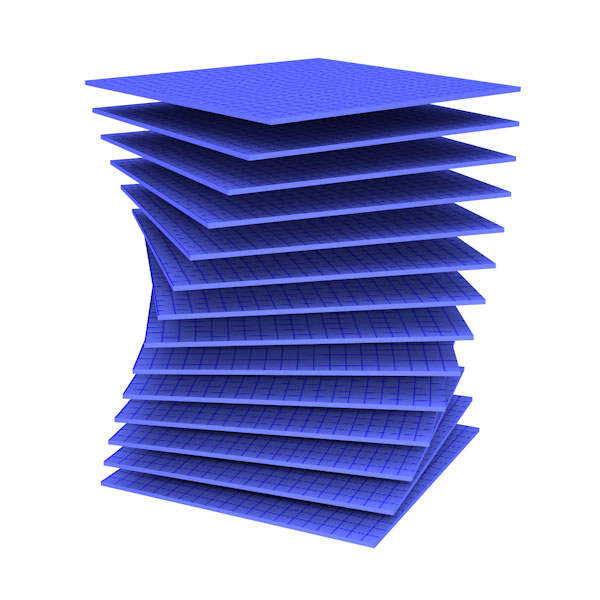This extra assumption is called two-surface orthogonality.

The assumption that the waves repeat could mean that space extends infinitely far but the wave pattern repeats like the tiles on an inlaid floor – as in the following image, which shows tiles with a simple pattern that has nothing whatsoever to do with gravitational waves: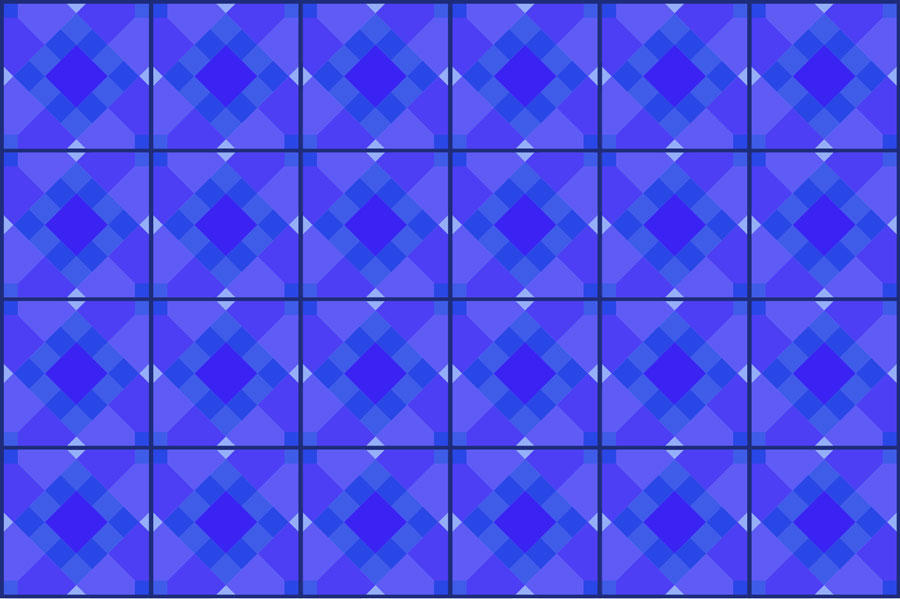Of course, the floor is a two-dimensional surface, while the repeating wave patterns fill three-dimensional space.

On such a tiled surface (or in such a tiled space), an object that crosses a boundary of a given tile just continues onto the next identical tile, as is the case with the moving red square in the following animation. On its round trip, the square visits four different tiles: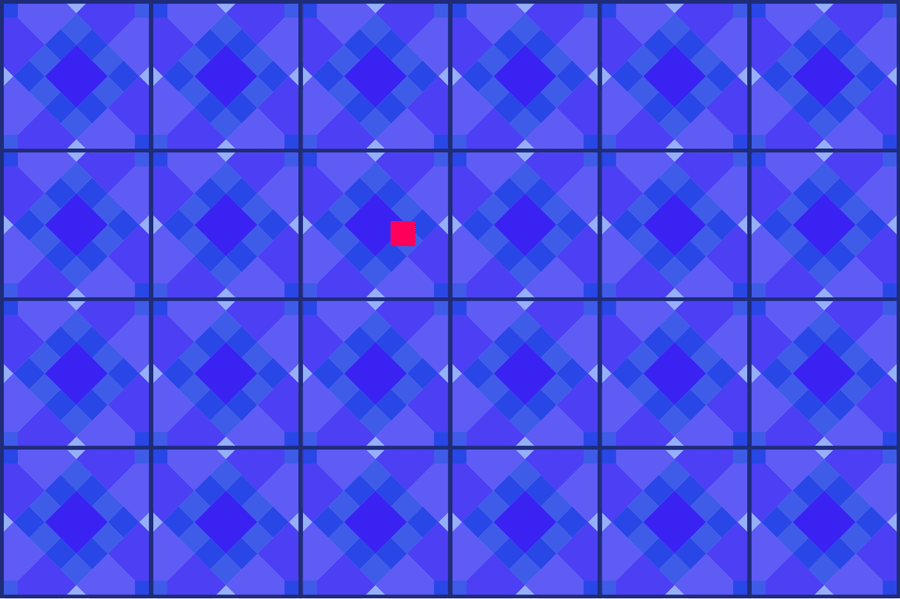But such perfect regularity could also be taken to mean that space itself is wrapped up so that any object leaving through one boundary of the “tile” will come back into that same tile through another boundary, as shown in this animation:If we are willing to distort the tile, it is possible to show directly how this new one-tile space looks like. Here is a simple tile without a pattern: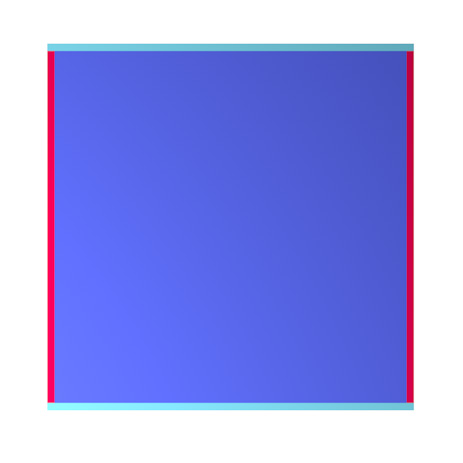We need to identify the two edges shown in cyan with each other; also, we need to identify the two edges shown in red. We can achieve identification by gluing the edges together. Gluing two opposite edges, the result is a cylindrical tube. Gluing the edges of the tube together, the result is a donut or torus: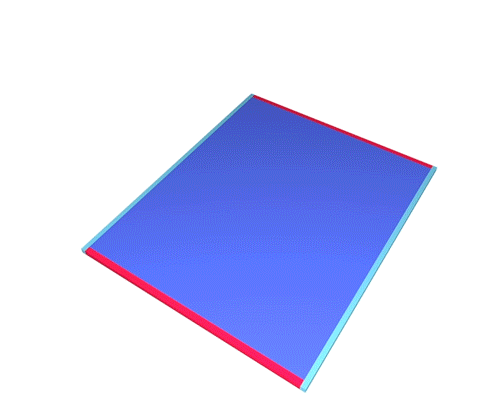For three-dimensional tiles, each of which is a solid cube, there is an analogous construction. The result is an object called a “three-dimensional torus”. On such a three-dimensional torus, the gravitational waves would simply chase each other around and around, similar to the way shown here for closed lines representing wave fronts on the surface of an ordinary two-dimensional torus: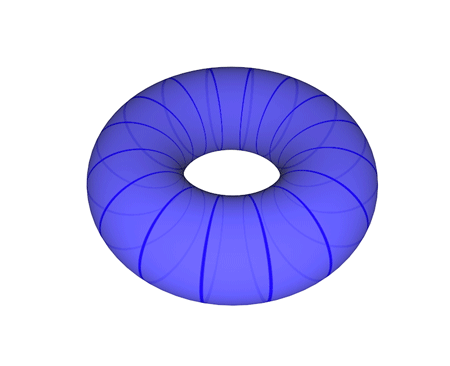For clarity, only waves moving around the torus in one direction are shown here. Actually Einstein’s equations tell us that, in these closed universes, there must always be waves moving in the opposite direction as well.

Given the two different interpretations of perfect regularity, whenever our third assumption holds, we can model two very different situations: an infinite universe with gravitational radiation that is uniformly distributed at large scales (all the tiles are the same) and a closed universe (just one tile). Our simplified model, no matter which way it is interpreted, is usually referred to as a Gowdy T3 spacetime, where T3 stands for the closed “three-dimensional torus” universe of just one tile.

When Einstein’s field equations are applied to this situation, they tell us right away that the evolution of these model universes is analogous to that of the more common big bang models: They have an abrupt and ill-defined beginning – an initial singularity -, and ever after, the whole universe keeps expanding.

At this stage, we can make one more simplifying assumption, namely

4. Only one of the two possible gravitational wave polarizations is present.

This amounts to saying that our gravitational waves are especially simple – the directions of maximal space distortion are the same for all of them. With this additional simplification, Einstein’s equations become so simple that they can actually be solved using pencil and paper: It is possible to write down a description for all possible spacetimes of this type using simple mathematical functions such as sine or cosine. Model universes that can simply be written down like this are called exact solutions of Einstein’s equations. The specific spacetimes discussed here are usually called “polarized Gowdy T3 spacetimes.”

In principle, we know everything there is to know about polarized Gowdy T3 spacetimes, so we can use them to test ideas about more general situations. For example, one might suspect that intense gravitational waves can lead to the formation of spacetime edges – singularities – that are not shielded from view by a black hole horizon. There is a conjecture stating that such naked singularities will not form (cosmic censorship). But in fact, in polarized Gowdy T3 spacetimes, no such naked singularities come into existence, so the conjecture remains safe from counter-examples.

Another conjecture called the BKL conjecture concerns the properties of spacetime near the initial singularity. It states that if you look at a small region of space, you will not be able to distinguish between this and almost all other spacetimes with an initial singularity. Near the singularity, all these universes show the same kind of behavior (see the spotlight Of singularities and breadmaking). That conjecture works for polarized Gowdy T3 spacetimes, so it too is still safe.

## Testing numerical simulations of gravitational wave sources

Another real situation that we are interested in understanding is the sort of gravitational wave train that is produced when two neutron stars or two black holes spiral into one another and coalesce. In that situation there is no symmetry and no way to come up with a “spherical chicken” model that will tell us much about the answer. We are forced to simulate the whole situation on a computer.

The computer uses a finite set of numbers to describe a continuous infinity of points. Since that is a perilous and error-prone procedure, we need some way to check it. The Gowdy T3 spacetimes are not much like a pair of inspiralling neutron stars or black holes, but they do describe a complex situation that we understand completely. Thus, they can be used to validate computer simulation procedures. Typically, a simulation program will be given a snapshot of the geometry of space and time (“initial data”); it then uses Einstein’s equations to calculate how this given situation evolves over time. For a polarized Gowdy T3 spacetime, we can write down explicitly how any snapshot evolves with time, so we know what the right answer should be. If a computer simulation is started with initial data corresponding to a snapshot of a polarized Gowdy T3 spacetime and gives results that agree with the known solution, then it passes the test. The following image gives an example: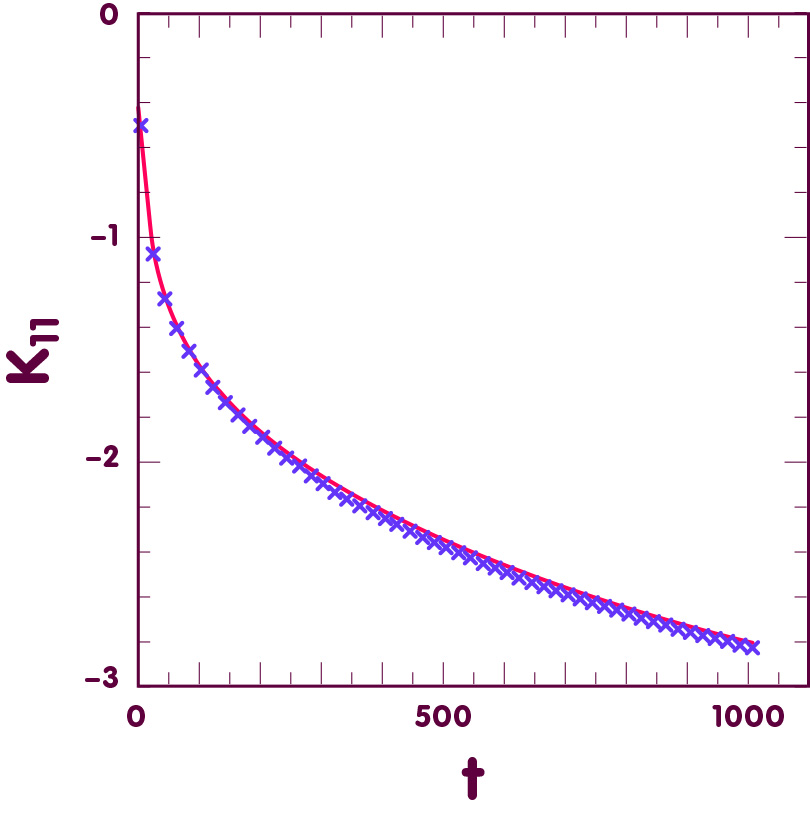[Image data from K. C. B. New et al., Phys.Rev. D58 (1998) 064022]

In this figure, physicists working on one particular simulation program have tracked the evolution of one particular quantity connected with the curvature of space called “K11“, comparing the results given by their program (violet crosses) with the known evolution in a polarized Gowdy T3 spacetime (red curve). As you can see, the computer program does rather well – for most of the time, you can hardly see the red curve behind the blue crosses; towards the end, however, the values given by the simulation are a tad smaller than the true values (the crosses are a bit below the red line).

## Focussed Gravitational Waves

Under suitable conditions, waves can diverge or converge (“come to a focus”). For light waves, a lens can produce this effect. For water waves, a stone thrown into a pond can create an “inverse focus point” from which the waves propagate outwards, seen here from above: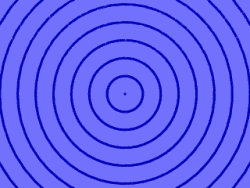The waves in a Gowdy T3 spacetime never come to a focus. But we might suspect that introducing focussed gravitational waves might lead to new phenomena. Indeed, if we replace the assumption of periodic waves with the assumption that the waves come to a (cylindrical) focus in two places, we can obtain another family of simple spacetimes whose properties can be worked out with pencil and paper. In these spacetimes, space is finite and curved on itself in one of two different generalizations of the familiar geometry of a sphere. (These solutions, Gowdy S¹xS² and Gowdy S³ spacetimes, really are “spherical chickens.”) The following animation shows a two-dimensional section of one of these spacetimes, including some of the gravitational wave fronts. As you can see, these waves are “emitted” at one focus point and travel along the sphere before being “absorbed” at another focus point: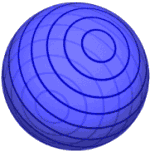However, this is again a simplified picture; in these spacetimes, just as in the case of the torus, there will be waves moving in the other direction as well.

The presence of places where the waves focus to a line makes these solutions somewhat more complex than the T3 spacetimes, so they have not been as fully explored, but it seems clear that they at least do not form naked singularities, so the cosmic censorship conjecture is safe from counterexamples here as well.

The remaining possibility is both very old and very new. If we replace the assumption of periodic waves with the assumption that the waves are cylindrical – focussed on just one line – two families of exactly solvable spacetimes result. One is the Einstein-Rosen cylindrical wave solution that was published in 1937 and formed the basis for early arguments about gravitational waves. It can be pictured as in the following animation, which shows a cubic region of space with expanding cylindrical wave fronts: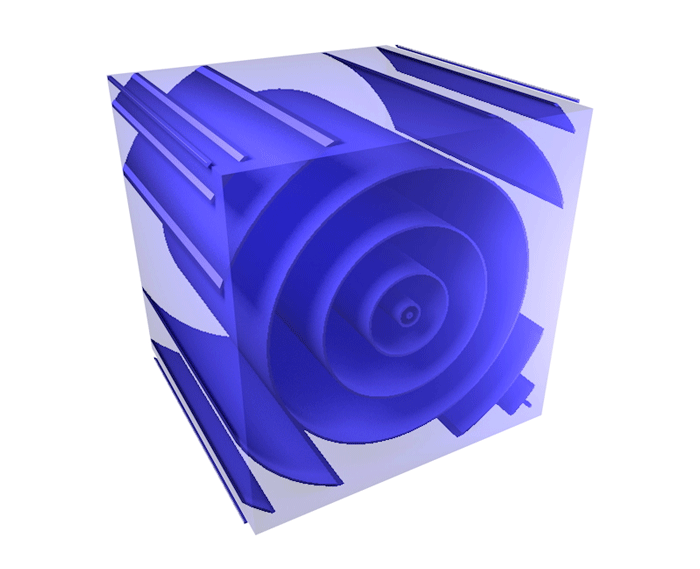The other spacetime corresponds to cylindrical waves in an expanding universe and was published in 2007. Both of these types of solutions can be used as simple models for gravitational waves that spread outward from a finite source such as a pair of inspiralling black holes.

## Interacting gravitational waves

All of the different assumptions – periodic waves, two-focus waves, and one-focus cylindrical waves – lead to exact, pencil and paper, solutions of Einstein’s field equations when only one polarization of gravitational wave is present. If we admit less simple gravitational waves by allowing the other polarization to be present, this results in what are called “unpolarized Gowdy spacetimes”. In contrast to the polarized case, it is impossible to write down a complete description of these spacetimes. Even so, they are comparatively simple in that they reduce Einstein’s complex field equations to much simpler equations for two interacting waves. These spacetimes offer a kind of “toy model” of Einstein’s theory of gravitation, with many of the complications removed. They can be used as a testing ground for understanding what Einstein’s theory predicts in more realistic situations. For example, it is quite simple to write computer programs that simulate unpolarized Gowdy spacetimes and explore the ways that different gravitational wave polarizations interact. One intriguing result is that regular waves can interact to produce what have been called “spikes”.

The following animation is based on the results of a numerical simulation of an unpolarized Gowdy spacetime. It shows how the amplitude of one of the two interacting gravitational waves changes throughout space (more precisely, in one particular direction of space). In the animation, time is running backwards – the animation begins at some particular moment in time and then follows the evolution of the expanding Gowdy universe backwards, coming ever closer to the initial singularity: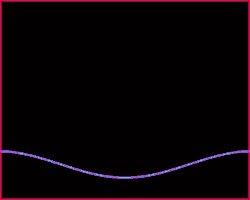[Animation: D. Garfinkle, Oakland University]

In the context of polarized Gowdy spacetimes, above, I had already mentioned the BKL conjecture, which predicts that the initial singularity should have a boring sameness everywhere – near that singularity, all regions of space should have approximately the same properties. As the animation shows, that is not quite true. Instead, the wave amplitude develops “spikes,” sharply defined regions of maximum and minimum amplitude. For those regions, the BKL conjecture fails. Because of this sort of counterexample, the BKL conjecture is now only thought to be true “almost everywhere,” failing at isolated points such as the locations of these spikes.

All in all, Gowdy spacetimes open up a variety of opportunities to explore the structure of Einstein’s universe – a testament to the usefulness of spherical chickens.

## Further Information

For the relativistic ideas behind this spotlight topic, check out Elementary Einstein, especially the chapters General Relativity and Gravitational waves.

Related Spotlights on relativity can be found in the sections General relativity and Gravitational waves.

###### Colophon
Robert H. Gowdy

is an Associate Professor of Physics at Virginia Commonwealth University with a research focus on the geometrical properties of spacetimes.